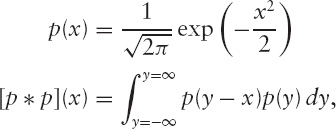# Exercises

## Exercise 1 (Section 2.1)

Ifwhat is f−∞ p(x) dx ? Then what isx,x2, andexp(x)?

## Exercise 2 (Section 2.1)

Show that the convolution of two Gaussian distributions,is also a Gaussian.

## Exercise 3 (Section 2.1)

Given the sets of random variablesz1= 0,z2= 0,

varz1 = 1,

varz

Get The Mathematics of Derivatives: Tools for Designing Numerical Algorithms now with the O’Reilly learning platform.

O’Reilly members experience live online training, plus books, videos, and digital content from nearly 200 publishers.